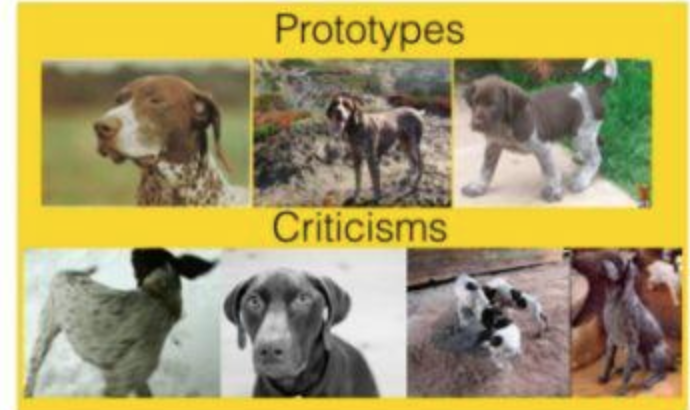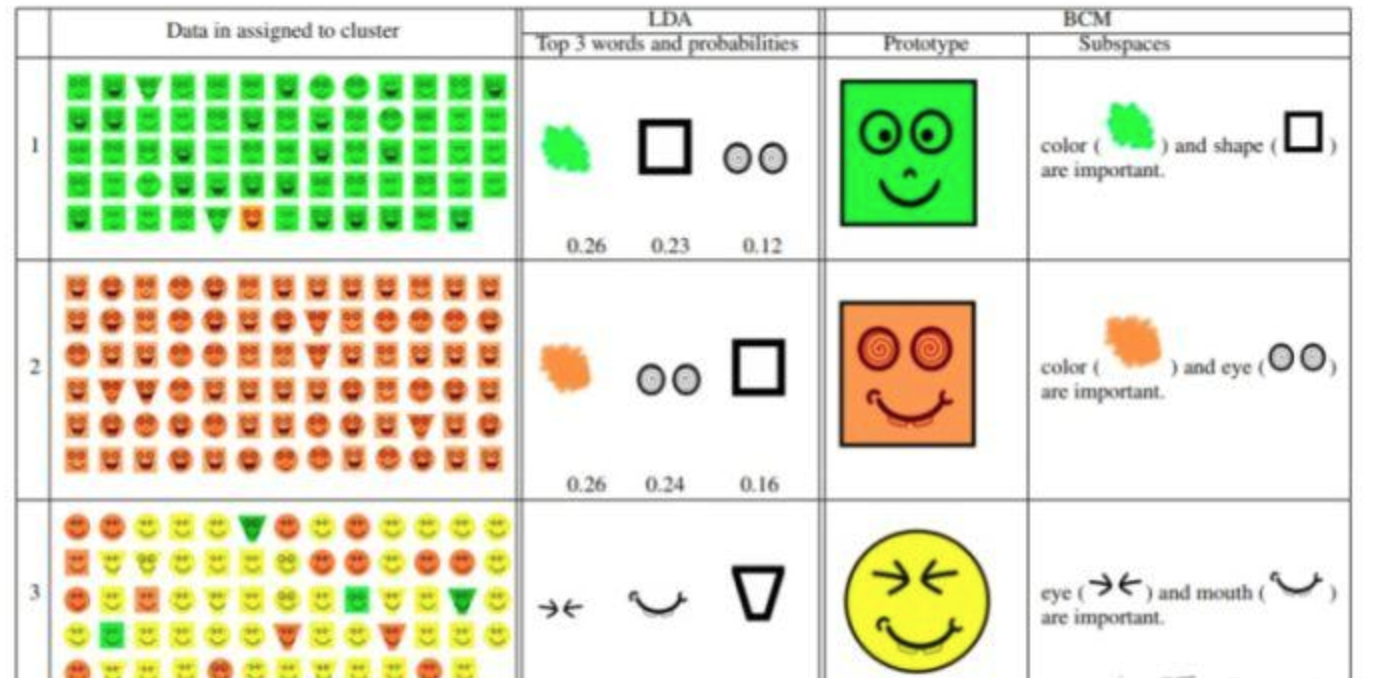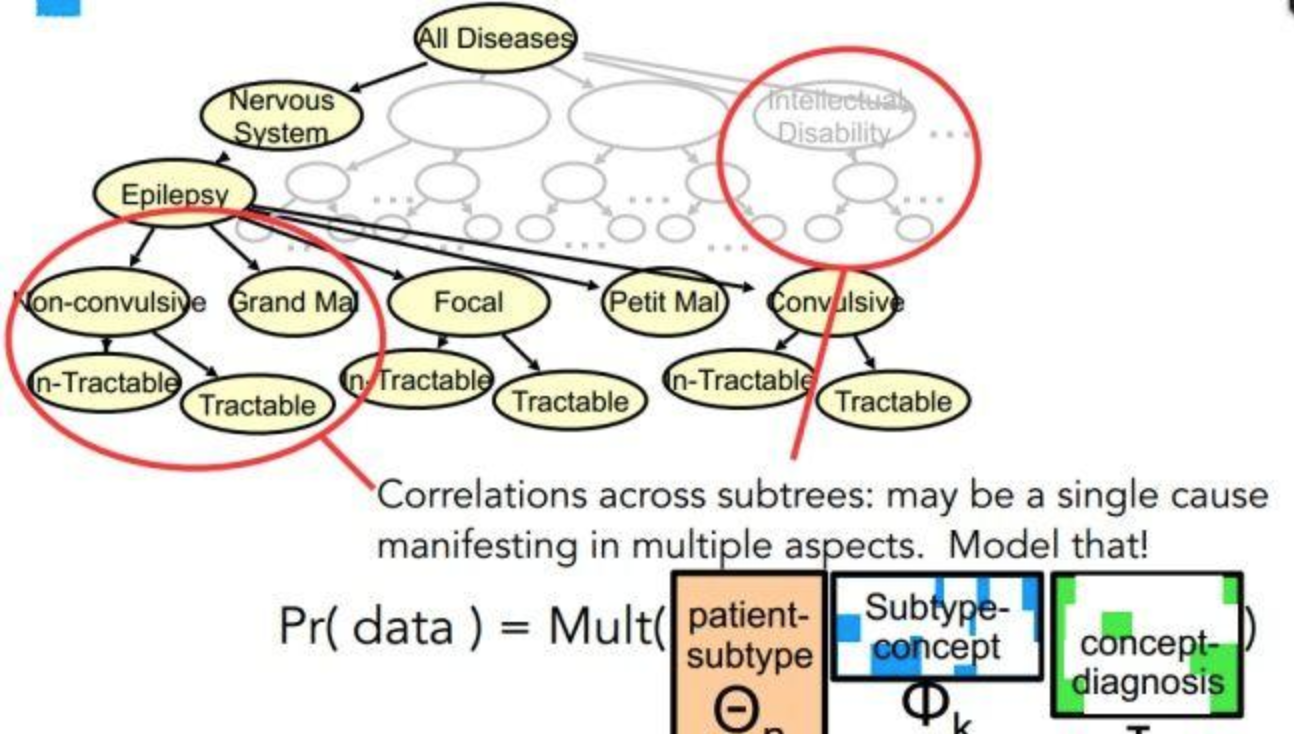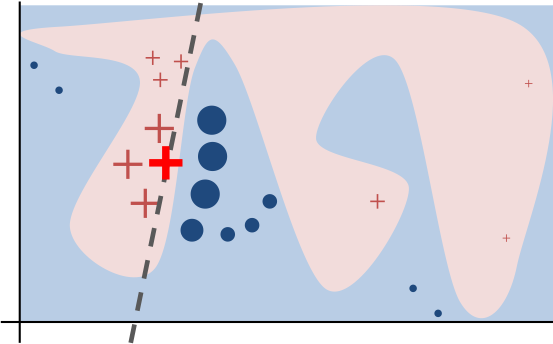# 1. 可解释性是什么

## 0x1：广义可解释性

在我们需要了解或解决一件事情的时候，我们可以获得我们所需要的足够的可以理解的信息。

# 2. 我们为什么需要可解释性？

## 0x1：可解释性的不同动机

### 1. 判别并减轻偏差（Identify and mitigate bias）

1. 数据集的规模可能有限，并且不能代表所有数据。
2. 或者数据捕获过程可能没有考虑到潜在的偏差。

## 0x2：在安全攻防AI 领域同样需要可解释性

### 2. 找到迭代优化的最快方向

https://www.leiphone.com/news/201805/Ti3mOdeRlWTplIlZ.html
https://cloud.tencent.com/developer/article/1096716
https://zhuanlan.zhihu.com/p/38151985
https://www.jiqizhixin.com/articles/2018-01-10

# 3. 有哪些可解释性方法

1. 在建模之前的可解释性方法
2. 建立本身具备可解释性的模型
3. 在建模之后使用可解释性方法对模型作出解释

## 0x1：在建模之前的可解释性方法

### 2. 样本数据探索## 0x2：建立本身具备可解释性的模型

1. 基于规则的方法（Rule-based）
2. 基于单个特征的方法（Per-feature-based）
3. 基于实例的方法（Case-based）
4. 稀疏性方法（Sparsity）
5. 单调性方法（Monotonicity）

### 3. 基于实例的方法（Case-based）### 4. 稀疏性方法（Sparsity）## 0x3：在建模之后使用可解释性方法对模型作出解释

1. 隐层分析方法
2. 模拟/代理模型
3. 敏感性分析方法

https://www.leiphone.com/news/201805/Ti3mOdeRlWTplIlZ.html
https://new.qq.com/omn/20180117/20180117A0T4JE.html 

# 4. Lime（local interpretable model-agnostic explanations）

Lime可通过可视化的方式向我们展示机器学习决策器是根据哪些“因素”进行了综合决策的。

## 0x1：Lime的原理## 0x2：通过Lime解释随机森林的决策因素

# -*- coding: utf-8 -*-

import lime
import sklearn
import numpy as np
import sklearn
import sklearn.ensemble
import sklearn.metrics

# For this tutorial, we'll be using the 20 newsgroups dataset. In particular, for simplicity, we'll use a 2-class subset: atheism and christianity.
from sklearn.datasets import fetch_20newsgroups
categories = ['alt.atheism', 'soc.religion.christian']
newsgroups_train = fetch_20newsgroups(subset='train', categories=categories)
newsgroups_test = fetch_20newsgroups(subset='test', categories=categories)
class_names = ['atheism', 'christian']

# Let's use the tfidf vectorizer, commonly used for text.
vectorizer = sklearn.feature_extraction.text.TfidfVectorizer(lowercase=False)
train_vectors = vectorizer.fit_transform(newsgroups_train.data)
test_vectors = vectorizer.transform(newsgroups_test.data)

# Now, let's say we want to use random forests for classification. It's usually hard to understand what random forests are doing, especially with many trees.
rf = sklearn.ensemble.RandomForestClassifier(n_estimators=500)
rf.fit(train_vectors, newsgroups_train.target)

pred = rf.predict(test_vectors)
res = sklearn.metrics.f1_score(newsgroups_test.target, pred, average='binary')

print res

# Explaining predictions using lime
from lime import lime_text
from sklearn.pipeline import make_pipeline
c = make_pipeline(vectorizer, rf)

print(c.predict_proba([newsgroups_test.data]))

# Now we create an explainer object. We pass the class_names a an argument for prettier display.
from lime.lime_text import LimeTextExplainer
explainer = LimeTextExplainer(class_names=class_names)

# We then generate an explanation with at most 6 features for an arbitrary document in the test set.
idx = 83
exp = explainer.explain_instance(newsgroups_test.data[idx], c.predict_proba, num_features=6)
print('Document id: %d' % idx)
print('Probability(christian) =', c.predict_proba([newsgroups_test.data[idx]])[0,1])
print('True class: %s' % class_names[newsgroups_test.target[idx]])

# The classifier got this example right (it predicted atheism).
# The explanation is presented below as a list of weighted features.
print exp.as_list()

# These weighted features are a linear model, which approximates the behaviour of the random forest classifier in the vicinity of the test example.
# Roughly, if we remove 'Posting' and 'Host' from the document , the prediction should move towards the opposite class (Christianity) by about 0.27 (the sum of the weights for both features).
# Let's see if this is the case.
print('Original prediction:', rf.predict_proba(test_vectors[idx])[0,1])
tmp = test_vectors[idx].copy()
tmp[0,vectorizer.vocabulary_['Posting']] = 0
tmp[0,vectorizer.vocabulary_['Host']] = 0
print('Prediction removing some features:', rf.predict_proba(tmp)[0,1])
print('Difference:', rf.predict_proba(tmp)[0,1] - rf.predict_proba(test_vectors[idx])[0,1])

# The explanations can be returned as a matplotlib barplot:
fig = exp.as_pyplot_figure()

# The explanations can also be exported as an html page (which we can render here in this notebook), using D3.js to render graphs.
exp.show_in_notebook(text=False)

# Alternatively, we can save the fully contained html page to a file:
exp.save_to_file('./oi.html')

# Finally, we can also include a visualization of the original document, with the words in the explanations highlighted. Notice how the words that affect the classifier the most are all in the email header.
exp.show_in_notebook(text=True)

https://github.com/marcotcr/lime
https://www.oreilly.com/learning/introduction-to-local-interpretable-model-agnostic-explanations-lime